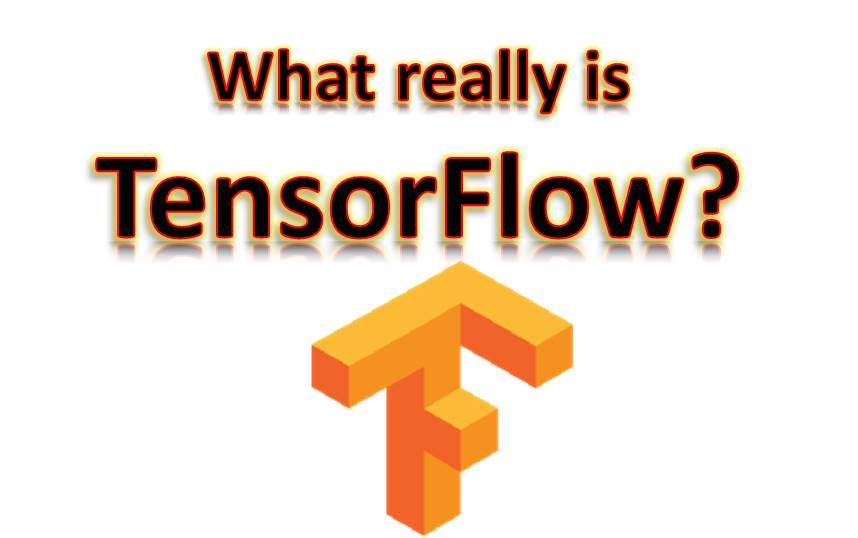# What the heck is TensorFlow (Beginner Tutorial 1)This would be a very easy explanation of the concept of TensorFlow. As you know, I’m Kindson the Genius

Sometimes, very simple terms may appear really complex due to the name. TensorFlow is a typical example. Although the underlying application of TensorFlow may be somewhat complex, that is Deep Learning. However TensorFlow is really an important tool you need to know and understand how it works.

In this brief article, I would clearly explain to you the concept of TensorFlow irrespective of whether you are an AI professional or not.
We would cover the following:
1. What is TensorFlow?
2. How to Use TensorFlow?
3. What is a Tensor?
5. Final Notes

### 1. What is TensorFlow?

TensorFlow is simple a tool developed by Google and used to perform computation.
But there is more.
TensorFlow is a library and can be imported and used with other programming languages. The typical and most common language used with TensorFlow is Python.
It is machine learning library and applies in neural network oriented problem

### 2. How to Use TensorFlow

TensorFlow is normally used with Python programming language. To use TensorFlow in Python, simply import the TensorFlow library into the session. This is the same way you add numpy or pandas to Python.
Once imported you can then use functions available in TensorFlow library.

### 3. What is a Tensor?

In the basic terms, Tensors are arrays used to hold data. But not just any array. Normally, very large high-dimension datasets which are in form of numbers are represented a tensors.
So we can say that the data we need to analyse a imported in TensorFlow as tensors.